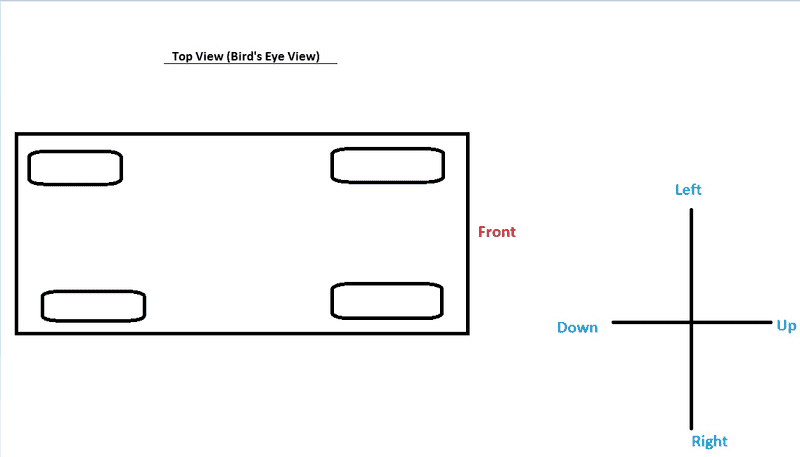# Car's weight shift with torque & angular momentum

I am having trouble visualizing which two tires of a car will be pushed down based on the angular momentum and torque of that car. Let's say if its angular momentum is point OUT while its torque is pointing UP in relations to the picture below.

My guess is it's the two right wheels of the picture below.

What I think is the angular momentum wants to go where the torque is in other words "the torque is pushing the angular momentum" you can figure out which way the car is leaning more and figure out which tires of the car carries the most weight at that point in time.

Picture: